# Purely Functional Left-Leaning Red-Black Trees

Kazu Yamamoto
created: 2011.10.19

We show that the idea of left-leaning reduces one pattern matching in the insertion operation of Okasaki's purely functional red-black trees. We proved in Coq that the invariants of left-leaning red-black trees stand for our purely functional algorithm of the insertion operation. Our benchmark shows that our algorithm is slightly faster than Okasaki's algorithm in some cases.

## History

In 1979, Guibas and Sedgewick published the original imperative red-black trees.

```Leo J. Guibas and Robert Sedgewick.
"A dichromatic framework for balanced trees",
In Proceedings of the 19th Annual Symposium on Computer Science,
pp8-21,
IEEE Computer Society,
1978
```

In this insertion algorithm, we need to take care of eight *unbalanced* cases. This algorithm is well described in "Introduction to Algorithms". Two can be reduced so six *unbalanded* cases are taken care of. If a professional programmer implements this algorithm, his Java code would be 150 lines. Sedgewick showed in "Algorithm in C" and "Algorithm in Java" that a tricky insertion can be written in 46 lines in Java.

In 1993, Andersson introduced the idea of right-leaning (the difference between right and left does not matter) and showed the red-black tree can be simplified.

```Arne Andersson,
"Balanced search trees made simple",
In Proceedings of the 3rd Workshop on Algorithms and Data Structures,
pp290-306.
1993
```

In 1999, Okasaki showed that the insertion operation can be implemented elegantly in the purely functional way. Its *balance* function takes care of four unbalanced cases and one balanced default case.

```Chris Okasaki,
"Red-Black Trees in a Functional Setting",
Journal of Functional Programming, 9(4),
pp471-477,
July 1999
```

In 2003, Letouzey and Filliatre proved in Coq, not only for the insertion(add) operation of Okasaki but also the delete(remove) operation.

```C. Filliatre and P. Letouzey,
"Functors for Proofs and Programs",
In Proceedings of The European Symposium on Programming,
volume 2986 of Lecture Notes in Computer Science,
pages 370-384
April 2004.
```

In 2008, Sedgwick revisited Andersson's idea and discovered left-leaning red-black trees. The original left-leaning red-black trees allows nodes whose two children are red (that is, this algorithm is another representaion of 2-3-4 trees). In the current left-leaning red-black trees, no red right child is allowed(that is, this algorithm is another representaion of 2-3 trees). This insertion algorithm is just 33 lines in Java.

```Robert Sedgewick
Left-leaning Red-Black Trees
2008
(Work in progress)
http://www.cs.princeton.edu/~rs/talks/LLRB/LLRB.pdf
http://www.cs.princeton.edu/~rs/talks/LLRB/RedBlack.pdf
```

## Purely Functional Red-Black Trees

The data structure of red-black trees can be defined as follows:

```data RBTree a = Leaf | Fork Color (RBTree a) a (RBTree a)
data Color = R | B
```

Invariants of red-black trees are as follows:

• Invariant 1. Every red node have two black children.
• Invariant 2. Every path from the root to a leaf contains the same number of black nodes.

Note that the color of the root is black and the color of leaves is also black.

We insert a new element as a red node. During insertion, a red node might have a red child. This means that balance is broken. We need to re-balance it. The number of such unbalanced cases is just four.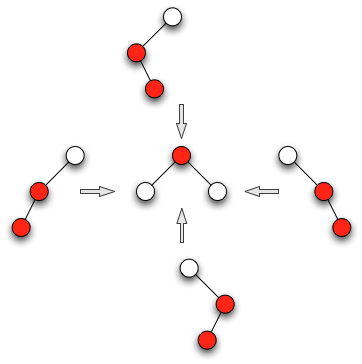With pattern match, this re-balancing is elegantly implemented as Okasaki discovered.

```insert :: Ord a => a -> RBTree a -> RBTree a
insert a b = Fork B d e f
where
Fork _ d e f = ins a b
ins x Leaf = Fork R Leaf x Leaf
ins x t@(Fork c l y r) = case compare x y of
LT -> balance c (ins x l) y r
GT -> balance c l y (ins x r)
EQ -> t

balance :: Color -> RBTree a -> a -> RBTree a -> RBTree a
balance B (Fork R (Fork R a x b) y c) z d = Fork R (Fork B a x b) y (Fork B c z d)
balance B (Fork R a x (Fork R b y c)) z d = Fork R (Fork B a x b) y (Fork B c z d)
balance B a x (Fork R b y (Fork R c z d)) = Fork R (Fork B a x b) y (Fork B c z d)
balance B a x (Fork R (Fork R b y c) z d) = Fork R (Fork B a x b) y (Fork B c z d)
balance k a x b = Fork k a x b
```

Exercise 3.9 of "Purely Functional Data Structures" suggests that we can avoid unnecessary pattern matching by dividing the balance function as follows:

```insert :: Ord a => a -> RBTree a -> RBTree a
insert a b = Fork B d e f
where
Fork _ d e f = ins a b
ins x Leaf = Fork R Leaf x Leaf
ins x t@(Fork c l y r) = case compare x y of
LT -> balanceL c (ins x l) y r
GT -> balanceR c l y (ins x r)
EQ -> t

balanceL :: Color -> RBTree a -> a -> RBTree a -> RBTree a
balanceL B (Fork R (Fork R a x b) y c) z d = Fork R (Fork B a x b) y (Fork B c z d)
balanceL B (Fork R a x (Fork R b y c)) z d = Fork R (Fork B a x b) y (Fork B c z d)
balanceL k a x b                           = Fork k a x b

balanceR :: Color -> RBTree a -> a -> RBTree a -> RBTree a
balanceR B a x (Fork R b y (Fork R c z d)) = Fork R (Fork B a x b) y (Fork B c z d)
balanceR B a x (Fork R (Fork R b y c) z d) = Fork R (Fork B a x b) y (Fork B c z d)
balanceR k a x b                           = Fork k a x b
```

## Purely Functional Left-Leaning Red-Black Trees

Left-leaning red-black trees introduce an additional invariant.

• Invariant 3. No red right child exists.

The following figure illustrates Invariant 3: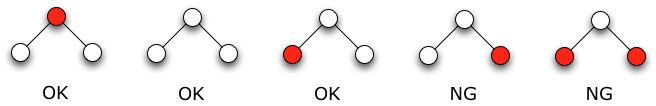In "Efficient Verified Red-Black Trees", Appel says:

```My student Max Rosmarin (Rosmarin, 2011) studied the question
of whether using the left-leaning invariant would mix well
with the Okasaki-style functional program, so as to factor the
implementations and proofs. Rosmarin demonstrated that Okasaki's
balance function can be factored into Sedgewick's three
operations. Although it is not conceptually more complex, the
factored function has more lines of code. Recall that Okasaki's
function, as I presented it here, has only 10 lines, which
is hard to improve on.
```

But we believe that we can reduce one pattern matching from Okasaki's insertion with the idea of left-leaning. The Sedgewick's implementation of left-leaning red-black trees in Java is as follows:

```private Node insert(Node h, Key key, Value val) {
if (h == null)
return new Node(key, val, RED);

int cmp = key.compareTo(h.key);

if (cmp == 0)
h.val = val;
else if (cmp < 0)
h.left = insert(h.left, key, val);
else
h.right = insert(h.right, key, val);

if (isRed(h.right))
h = rotateLeft(h);

if (isRed(h.left) && isRed(h.left.left))
h = rotateRight(h);

if (isRed(h.left) && isRed(h.right))
colorFlip(h);

return h;
}
```

14 October 2011, Yamamoto found that this code can be implemented in purely functional manner as follows:

```insert :: Ord a => a -> RBTree a -> RBTree a
insert a b = Fork B d e f
where
Fork _ d e f = ins a b
ins x Leaf = Fork R Leaf x Leaf
ins x t@(Fork c l y r) = case compare x y of
LT -> balanceL c (ins x l) y r
GT -> balanceR c l y (ins x r)
EQ -> t

balanceL :: Color -> RBTree a -> a -> RBTree a -> RBTree a
balanceL B (Fork R (Fork R a x b) y c) z d = Fork R (Fork B a x b) y (Fork B c z d)
balanceL k a x b                           = Fork k a x b

balanceR :: Color -> RBTree a -> a -> RBTree a -> RBTree a
balanceR B (Fork R a x b) y (Fork R c z d) = Fork R (Fork B a x b) y (Fork B c z d)
balanceR k x y (Fork R c z d)              = Fork k (Fork R x y c) z d
balanceR k a x b                           = Fork k a x b
```

Note that the number of pattern matching is reduced by one and one pattern matching is shallower.

When inserting a red node to left, one unbalance case would happen. In this case, balance is recovered like Okasaki's algorithm. Note that three of the four unbalanced cases of Okasaki do not happen thanks to Invariant 3.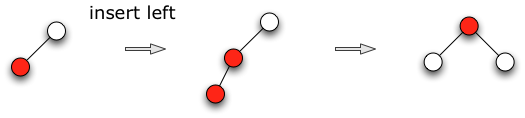When inserting a red node to right, three cases happen which break Invariant 3. Two of the three cases, which are marked with the orange star, can be implement with one pattern match.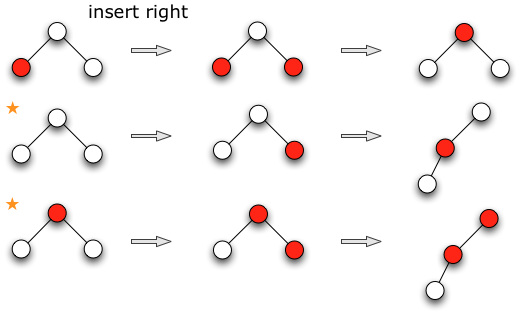18 October 2011, Hirai proved in Coq the three invariants stand for this insertion algorithm.

## Benchmark

Normalizing the results of left-leaning red-black trees by that of Okasaki's red-black trees.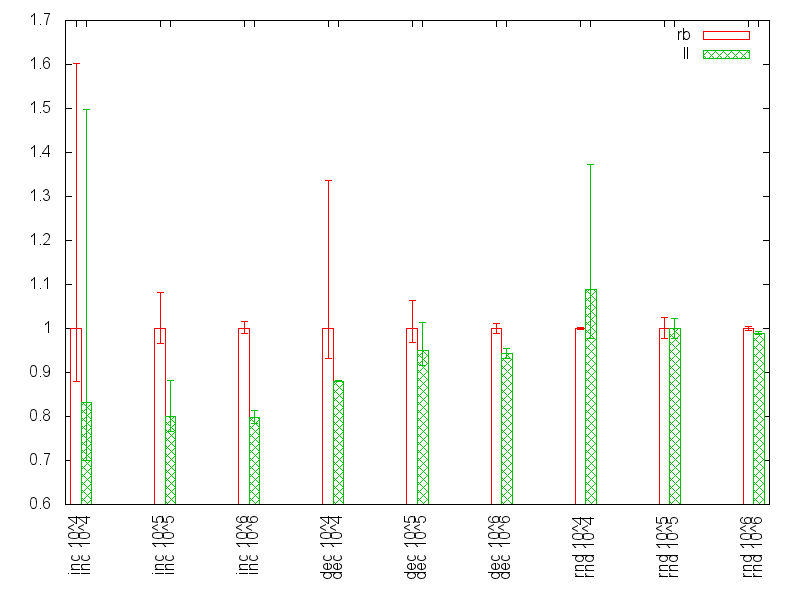• Left-leaning is faster in increasing input
• Left-leaning is slightly faster in decreasing input
• Left-leaning is almost the same in random input